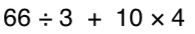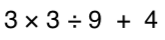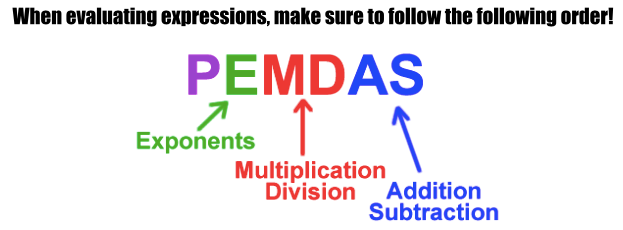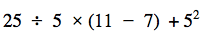Integers
Order of Operations
One-Step Equations
Distributive Property
Two-Step Equations
100

What is (-100) + (+25)=

-75

100

Simplify the following expression:100

5x = 60

What is x = 12

100

8(4+2) = ?

What is 48?

100

2k + 5 = 17

What is 6 ?

200

What is (-224) - (-25)=

-199

200

Simplify the following expression:200

-0.3m = -12

What is 40?

200

5(x-3)

What is 5x-15?

200

5m - 7 = 23

What is 6?

300

What is (+9) x (-8)=

-72

300

3 + 42 * (3 + 2)What is 95?

300

x + 8 = -60

What is -68

300

7+9(2x-3)

What is 18x-20?

300

12s + 27 = 171

What is 12 ?

400

What is (-56)/(+8)=

-7

400What is 45?

400

x - (-3) = 5

What is 2

400

-3(-x-10)

What is 3x+30?

400

19b + 314 = 1663

What is 71 ?

500

What are positive and negative numbers called?

Integers

500What is 30?

500

x - 0.9x = 7

What is 70?

500

x + 10 -6(x-3x) +4

What is 13x+14?

500

4j - 71 = 29

What is 25 ?

Click to zoom